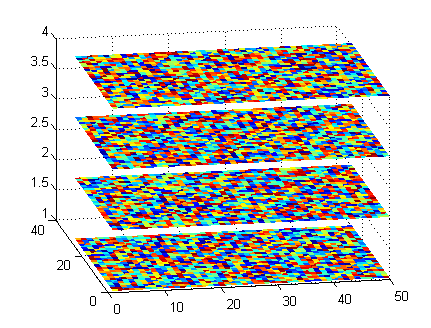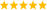LabVIEW

cancel
Showing results for
Did you mean:

Highlighted

plotting a 4D graph from a 3D array

Hi

I currently have a 3D array ( X,Y,Z) . where  2D intensity plane values are stored within a Z value of the array. I would like to plot a stack of these images in 3D in labview. where X,Y are of the intensity coordinates, I is the intensity value and Z is the spacing between corresponding planes. I want like the plot to look something like this:I have seen previous examples of people doing this with CWgraph3d function on the links below:

https://forums.ni.com/t5/LabVIEW/4d-plot-from-a-3d-array/m-p/1005924

https://forums.ni.com/t5/LabVIEW/Best-3D-visualization/m-p/1441338#M553345

I tried using this function, only to find out that it is only supported with the 32-bit version of Labview and not the 64-bit which I am currently using.

So I have tried playing  with the 3D parametric graph vi but so far no luck...

Message 1 of 13
(1,201 Views)

Re: plotting a 4D graph from a 3D array

Not sure if this is exactly what you are looking for but this may be useful:

http://www.ni.com/example/25468/en/

CH
Applications Engineering
National Instruments
http://www.ni.com/en-us/support.html
Message 2 of 13
(1,153 Views)

Re: plotting a 4D graph from a 3D array

@protocoled wrote:

Not sure if this is exactly what you are looking for but this may be useful:

http://www.ni.com/example/25468/en/

Judging by the date that example would be using the Component Works version which the OP said would not work for them.

Ben

Message 3 of 13
(1,150 Views)

Re: plotting a 4D graph from a 3D array

@zak9000 wrote:

I tried using this function, only to find out that it is only supported with the 32-bit version of Labview and not the 64-bit which I am currently using.

Do you have a good reason for using 64bit. You can easily install both and use 32bit LabVIEW for most things.LabVIEW Champion. It all comes together inGCentralMessage 4 of 13
(1,147 Views)

Re: plotting a 4D graph from a 3D array

Hi

I have some hardware peripheral that is only compatible with the 64-bit version. Anyhow I got it working now , it was simpler than I expected after I realized that the 3d surface graph had an extra node for a colourmap. Attached is an example of how i got it working.

Now I have a stack of intensity based images and was wondering if it would be possibe to interpolate between the images to fill the gaps and also currently my x and y are 1D arrays whereas by z axis is the 2D colour map but i was wondering if it would be possible to have x and z as 1D arrays and y as my 2D colour map. I played around with the graph settings to see if i could change z and y axis on the plot but the option was not there..

Message 5 of 13
(1,138 Views)

Re: plotting a 4D graph from a 3D array

I have little experience with the new non-CW version of the 3D graphs so the following may not apply to the new versions.

It all depends on how you present that data to plot. Interpolation was only valid in a plot and not between plots. So you have to process your data to put in a form that the 3D graph can understand.

If you have enough data points it is possible to  "cheat" and let the human eye do the interpolation. Another approach could do 3D binning where values from multiple plots are grouped into a single plot.

Ben

Message 6 of 13
(1,125 Views)

Re: plotting a 4D graph from a 3D array

Unfortunately, the 3D graphs, as far as I know, only allows the Z axis to be intensity. I don't think there are property settings that can change this. To do what you want to do, you will need to create a 3D Picture in LabVIEW which is more complicated. There are a few examples in the example finder that may be useful to look at.

CH
Applications Engineering
National Instruments
http://www.ni.com/en-us/support.html
Message 7 of 13
(1,117 Views)

Re: plotting a 4D graph from a 3D array

@protocoled wrote:

Unfortunately, the 3D graphs, as far as I know, only allows the Z axis to be intensity.

If you place a parametric surface graph on the front panel, you get a tool on the diagram that allows for an (optional) independent color in addition to xyz.I use it in one of my public programs to graph spherical harmonics. Each surface point has a x, y, z, and color.LabVIEW Champion. It all comes together inGCentralMessage 8 of 13
(1,109 Views)

Re: plotting a 4D graph from a 3D array

@altenbach wrote:

@protocoled wrote:

Unfortunately, the 3D graphs, as far as I know, only allows the Z axis to be intensity.

If you place a parametric surface graph on the front panel, you get a tool on the diagram that allows for an (optional) independent color in addition to xyz.I use it in one of my public programs to graph spherical harmonics. Each surface point has a x, y, z, and color.That is real pretty Christian.

I have not had a project that use 3D graphing lately so I am curious...

About how many data points involved in that image and how is the performance?

Am I correct that the assuming the XYZ matrix is define the surface on which the W values are being plotted?

Like I said, curious,

Ben

Message 9 of 13
(1,102 Views)

Re: plotting a 4D graph from a 3D array

@Ben wrote:
About how many data points involved in that image and how is the performance?

Performance is interactive (recalculations and display) and basically instantaneous. The 2D arrays are 128x128, sufficient for visualization. No results are used in later calculations.

Yes, w is the color and xyz define the sphere.LabVIEW Champion. It all comes together inGCentralMessage 10 of 13
(1,094 Views)